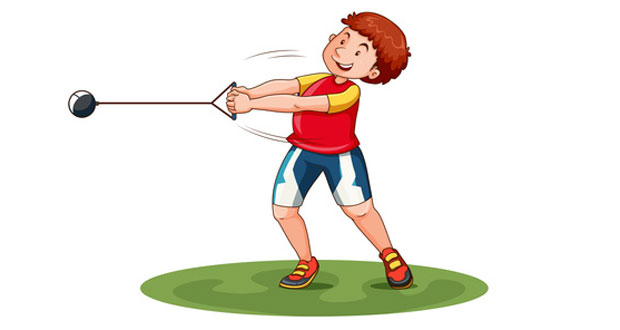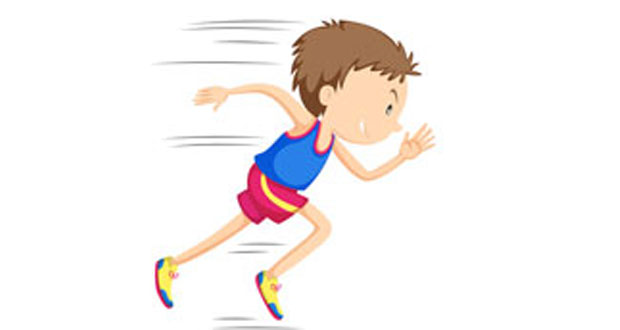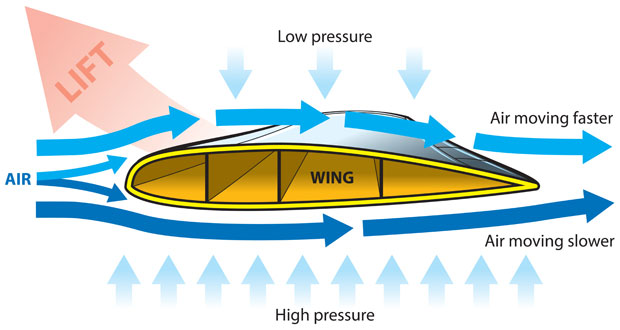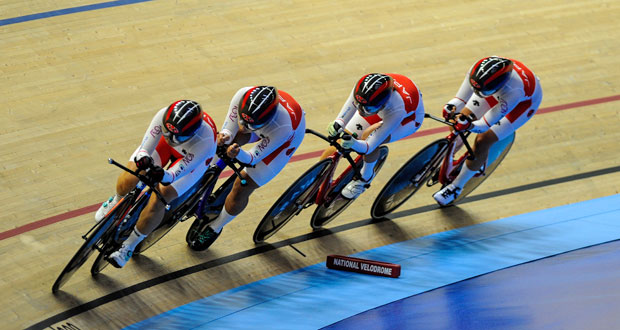# Biomechanics

## Angular Motion In BiomechanicsAngular motion includes rotating bodies, levers, stability, moment of force/torque, axis of rotation, moment of inertia and angular momentum. Lever Systems A lever is a rigid bar that moves on a fixed point called the fulcrum when a force is applied to it. Movement is made possible in the human body by lever systems that …

## Linear Motion & BiomechanicsLinear motion simply means motion in a straight line (as opposed to circular motion or rotation). In order to talk about linear motion scientifically, we need to be familiar with mass, distance, displacement, speed, velocity, and acceleration. Here we explain Newton’s laws of motion, mass, inertia, momentum, speed, velocity, distance, displacement and graphs of motion. …

## Bernoulli PrincipleThe Bernoulli principle explains why aeroplanes fly and racing cars stick to the ground going around corners when they really shouldn’t. It is to do with lift force and downforce created when air flows over an aerofoil. The Dutch-Swiss mathematician, Daniel Bernoulli, discovered that where there is an increase in the velocity of fluid there …

## Air Resistance and DragAir resistance is a frictional force that occurs when air passes over the surface of a body. This frictional force is not just limited to air though and it applies in fluids, usually water in a sporting situation. Air resistance, drag, and fluid resistance mean the same thing. Four things affect the amount of air resistance; …

Scroll to Top